## Calibration parameters (校正參數) for drawing an SVG file Back

Here what I wrote is all about recording the calculation of the calibration parameters when drawing a SVG file. Before that, you're supposed to know how to draw a SVG file with canvas in JavaScript.

### Why need calibration parameters?

In the last step of drawing, we will find that there exists a problem. As shown below, there is a canvas area, which I call a panel.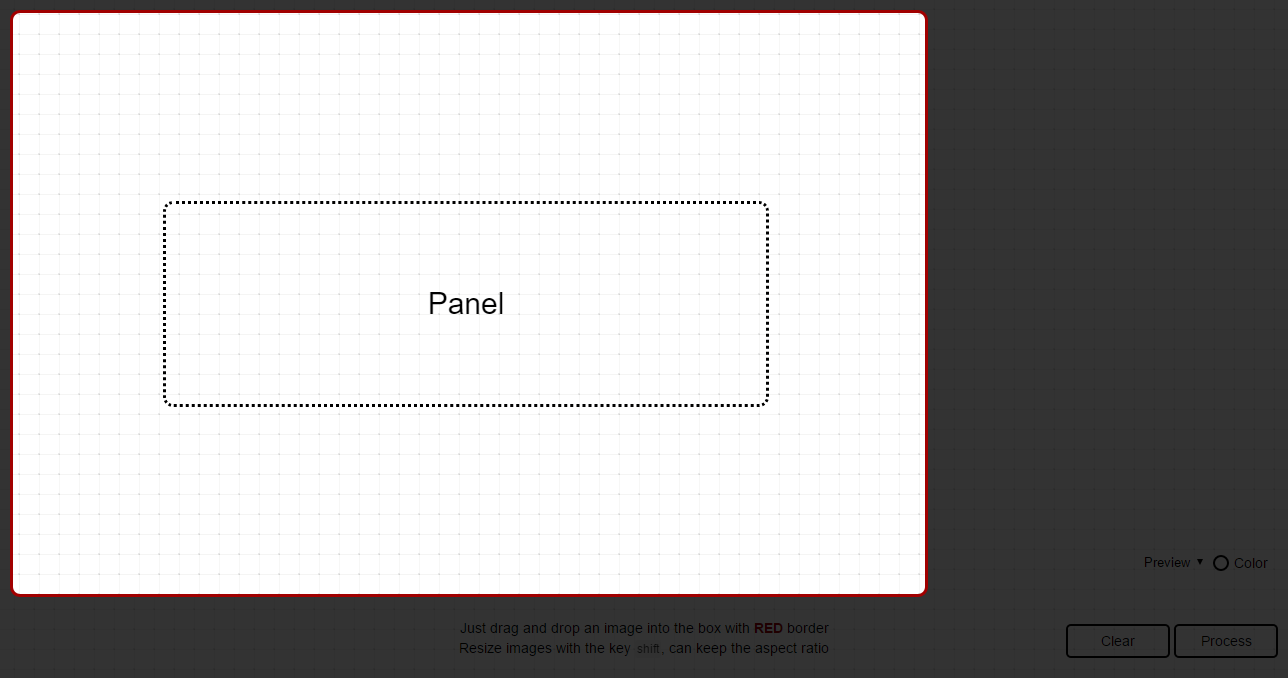When I drop a SVG file into this panel, and start to draw it,I find that only data of points is not enough, because the data should always depend on some attributes of a SVG element, including `width`, `height`, and `viewBox`. Besides, what if I can drag and resize this SVG like below: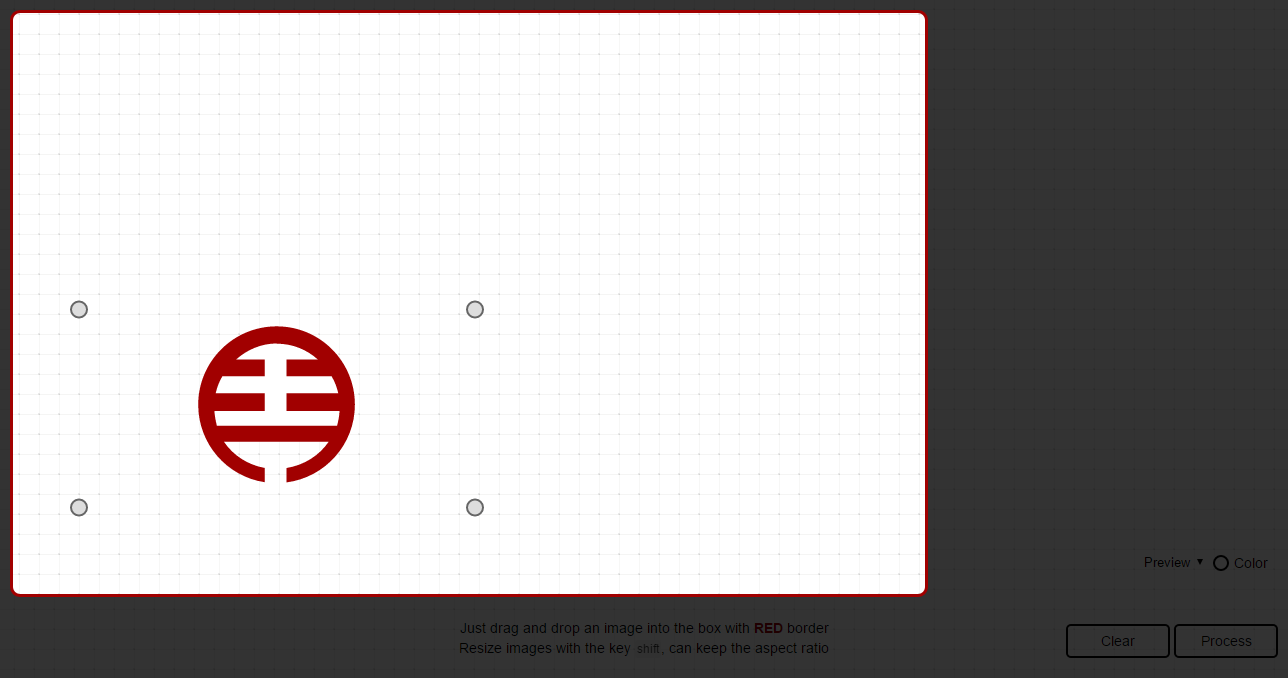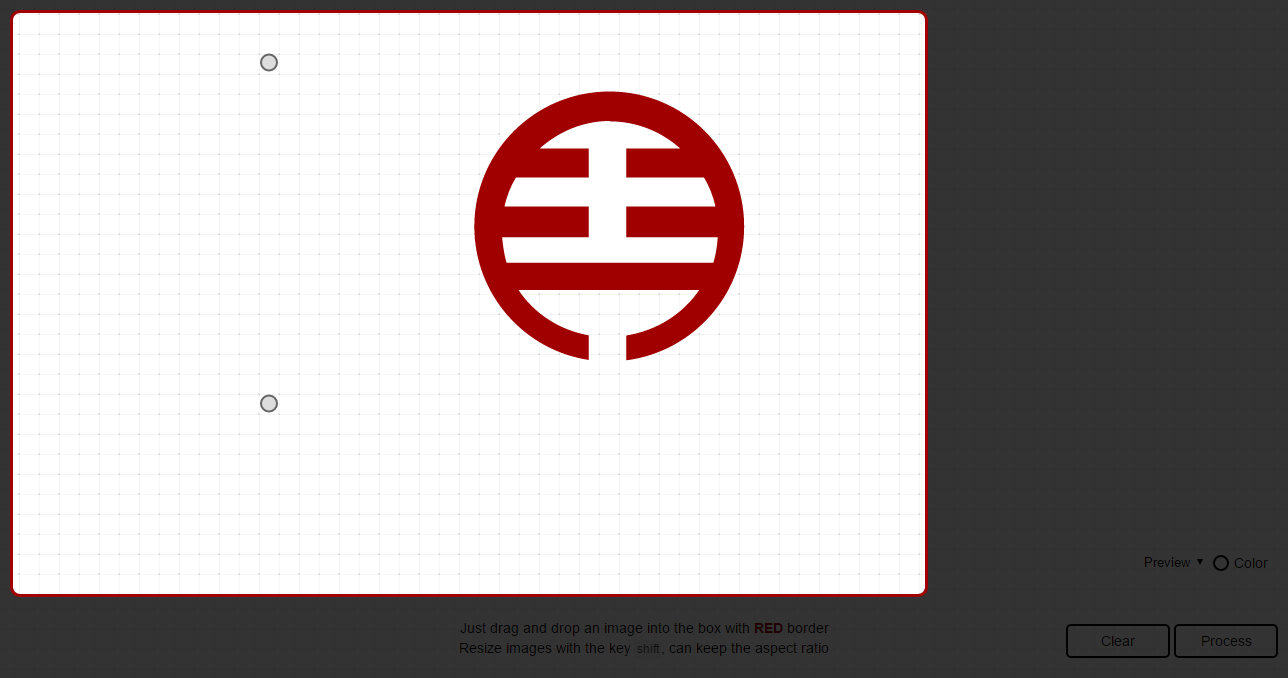As I change the size of this SVG, data of points in paths won't change at the same time. What that means is that what I have drawn should be always in the same position.

Attributes like `width`, `height`, and `viewBox` of this SVG element will also affect data of points like dragging.

``````<svg xmlns="http://www.w3.org/2000/svg" width="400" height="200" viewBox="0 0 200 200">
<!-- paths -->
</svg>
``````

That's why I need a calibration parameter for updating data of points in paths.

### Calculation

So I have defined some parameters: `dx`, `dy`, `ratioX`, and `ratioY`. Before calculation, there are some aspects needed to be explained.

• Group: a group for storing image and 4 respective circles, which is used for dragging to resize
• Image: the image stored in a group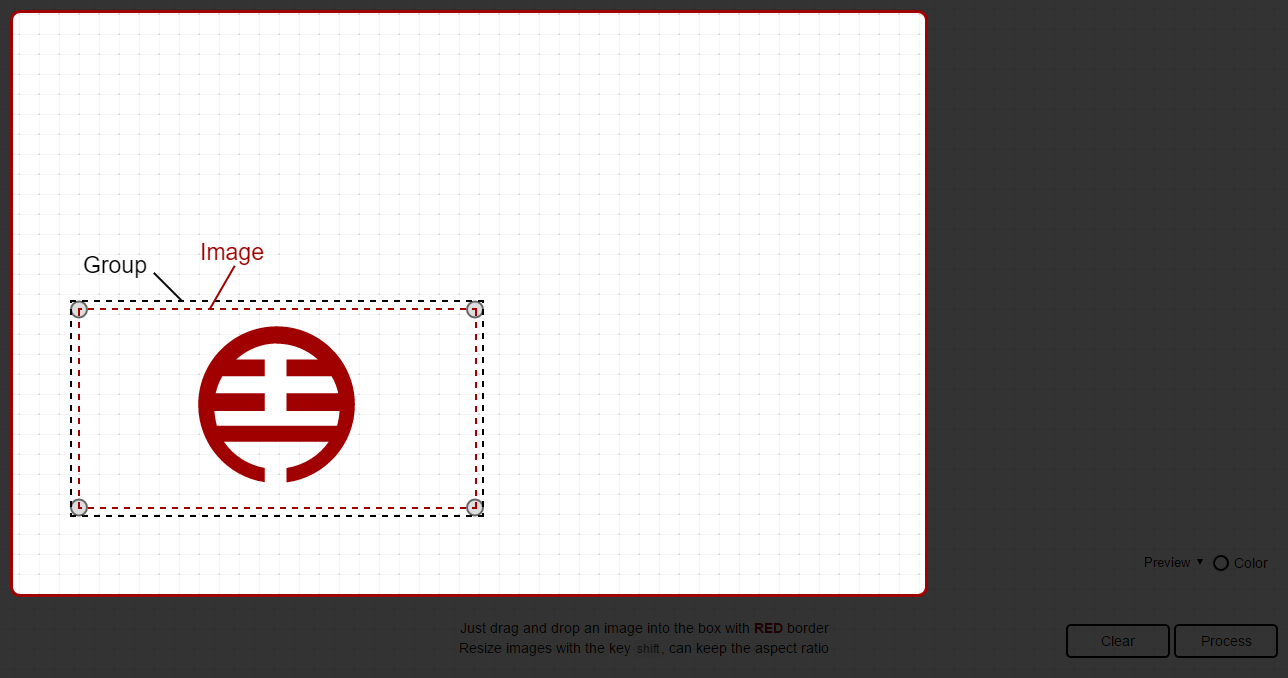#### Positions

`(dx,dy)` represent the current position of that image.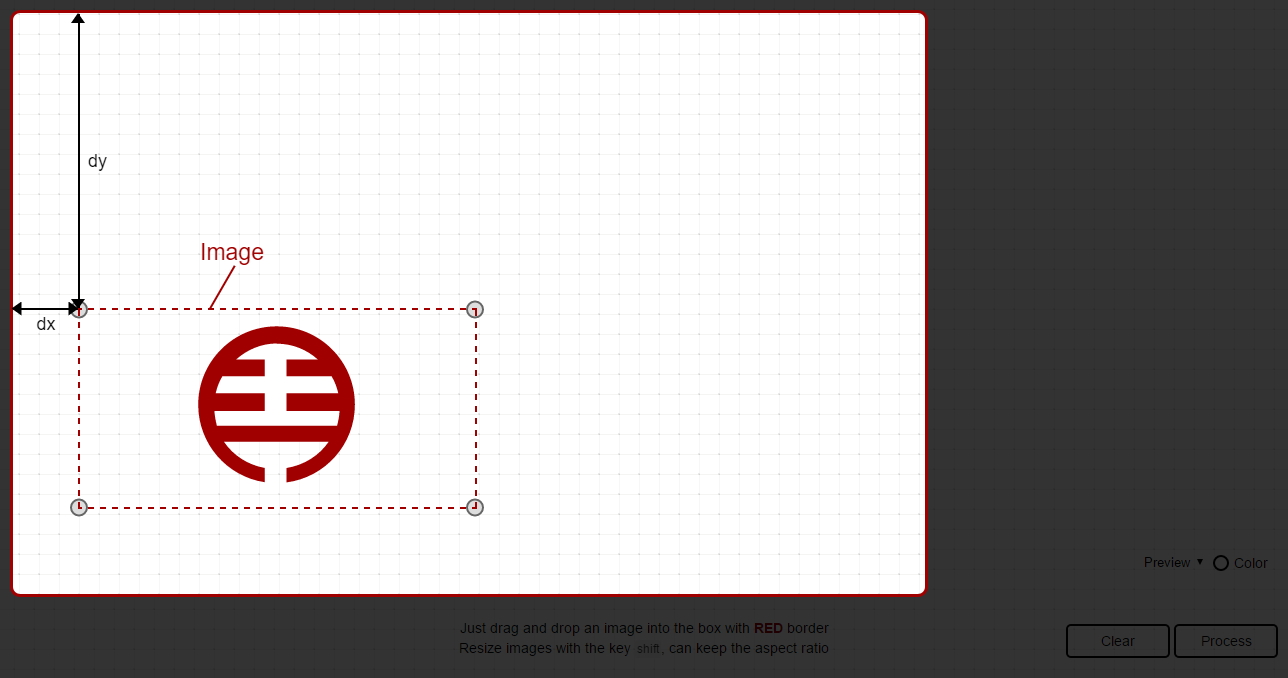• oriX: the original `x` value of the image
• oriY: the original `y` value of the image
• moveX: difference of the `x` value.
• moveY: difference of the `y` value.
• viewBoxX: the `x` value of the attribute `viewBox` of that image
• viewBoxY: the `y` value of the attribute `viewBox` of that image
``````var dx = image.oriX + image.moveX;
var dy = image.oriY + image.moveY;
``````

#### Ratio

Ratio is affected by some factors, and the `calibrationParam` is calculated as below:

• oriW: the original width of the image
• oriH: the original height of the image
• svgW: the width of the SVG element
• svgH: the height of the SVG element
• viewBoxW: the width of the attribute `viewBox` in that SVG element
• viewBoxH: the height of the attribute `viewBox` in that SVG element
• curW: the current width of the image
• curH: the current height of the image
``````var calibrationParam = Math.max(image.oriW / image.svgW, image.oriH / image.svgH) * Math.min(image.svgW / image.viewBoxW, image.svgH / image.viewBoxH);

var ratioX = (image.curW / image.oriW) * calibrationParam;
var ratioY = (image.curH / image.oriH) * calibrationParam;
``````

Note: remember that the `x` and `y` value of the attribute `viewBox` will also crop that SVG to show.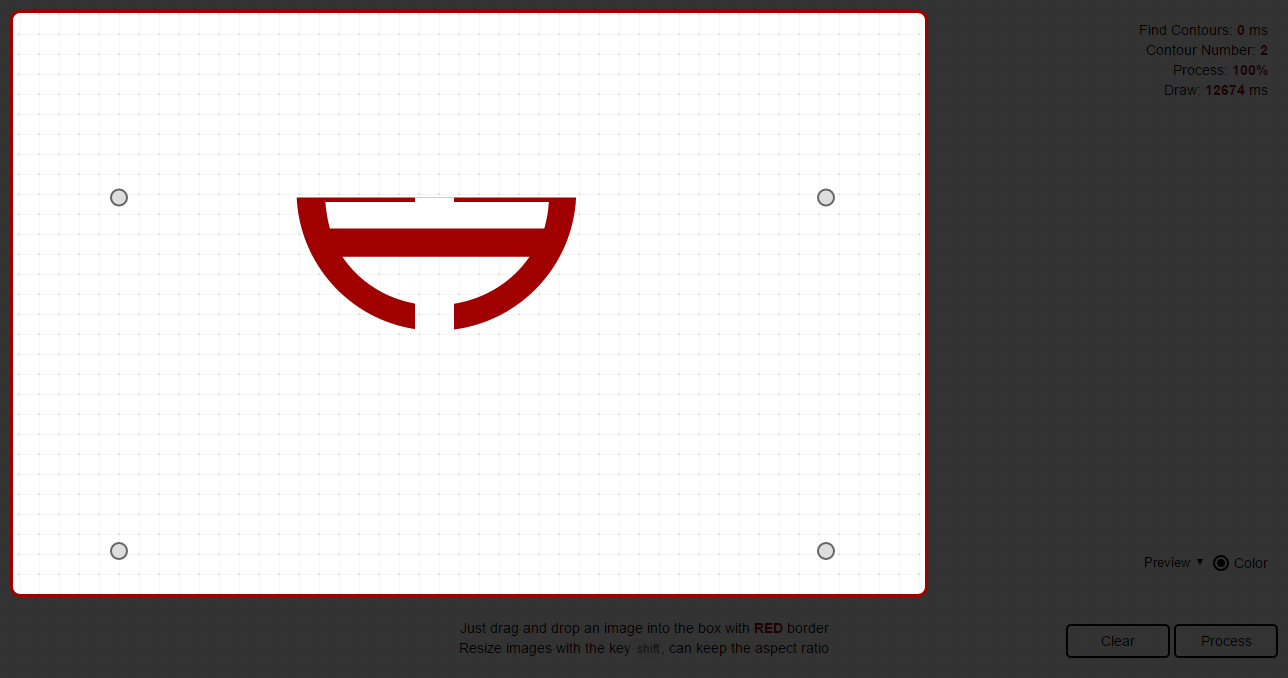For this reason, we should fill data of points before drawing like this:

``````var pointsArr = [];
var pathLen = pathNodes.length;

for (var j = 0; j < pathLen; j++) {
var index = pointsArr[].push([]);
var pointsLen = pathNodes[j].getTotalLength();

for (var k = 0; k < pointsLen; k++) {
/** extract points from a path */
var data = pathNodes[j].getPointAtLength(k);

/** calculation of calibration parameters */
var dx = image.oriX + image.moveX;
var dy = image.oriY + image.moveY;

var calibrationParam = Math.max(image.oriW / image.svgW, image.oriH / image.svgH) * Math.min(image.svgW / image.viewBoxW, image.svgH / image.viewBoxH);

var ratioX = (image.curW / image.oriW) * calibrationParam;
var ratioY = (image.curH / image.oriH) * calibrationParam;

data.x = data.x * ratioX + dx - image.viewBoxX;
data.y = data.y * ratioY + dy - image.viewBoxY;

/** filter for cropping SVG */
if (data.x >= dx &&
data.x <= dx + image.curW &&
data.y >= dy &&
data.y <= dy + image.curH) {
pointsArr[index].push(data);
}
}
}
``````

### Optimization

``````var pointsArr = [];
var pathLen = pathNodes.length;

for (var j = 0; j < pathLen; j++) {
var index = pointsArr[].push([]);
var pointsLen = pathNodes[j].getTotalLength();

for (var k = 0; k < pointsLen; k++) {
/** extract points from a path */
var data = pathNodes[j].getPointAtLength(k);

/** calculation of calibration parameters */
var dx = image.oriX + image.moveX;
var dy = image.oriY + image.moveY;

var calibrationParam = Math.max(image.oriW / image.svgW, image.oriH / image.svgH) * Math.min(image.svgW / image.viewBoxW, image.svgH / image.viewBoxH);

var ratioX = (image.curW / image.oriW) * calibrationParam;
var ratioY = (image.curH / image.oriH) * calibrationParam;

data.x = (data.x - image.viewBoxX) * ratioX + dx;
data.y = (data.y - image.viewBoxY) * ratioY + dy;

data.x = data.x >= dx && data.x <= dx + curW ? data.x : ((data.x < dx) ? dx : dx + curW);
data.y = data.y >= dy && data.y <= dy + curH ? data.y : ((data.y < dy) ? dy : dy + curH);

/** filter with edge */
pointsArr[index].push(data);
}
}
``````